# Describe Characteristics of a Parent Function Describe the

• Slides: 37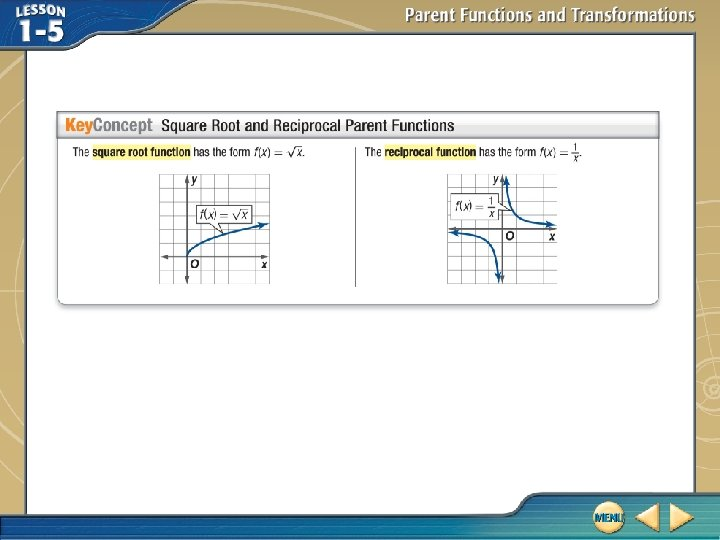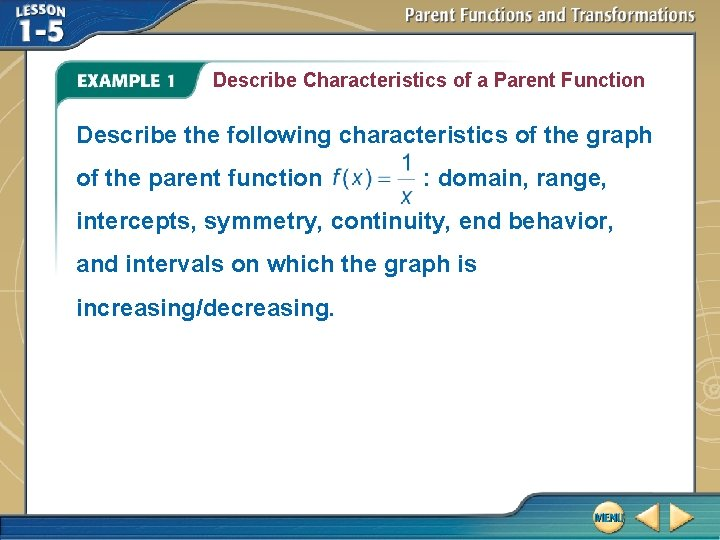Describe Characteristics of a Parent Function Describe the following characteristics of the graph of the parent function : domain, range, intercepts, symmetry, continuity, end behavior, and intervals on which the graph is increasing/decreasing.Describe Characteristics of a Parent Function The graph of the reciprocal function shown in the graph has the following characteristics. The domain of the function is, and the range is. The graph has no intercepts. The graph is symmetric with respect to the origin, so f (x) is odd.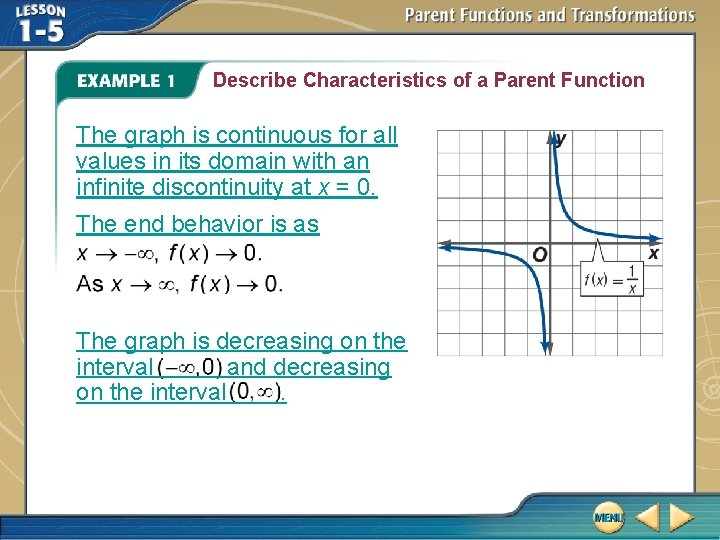Describe Characteristics of a Parent Function The graph is continuous for all values in its domain with an infinite discontinuity at x = 0. The end behavior is as The graph is decreasing on the interval and decreasing on the interval.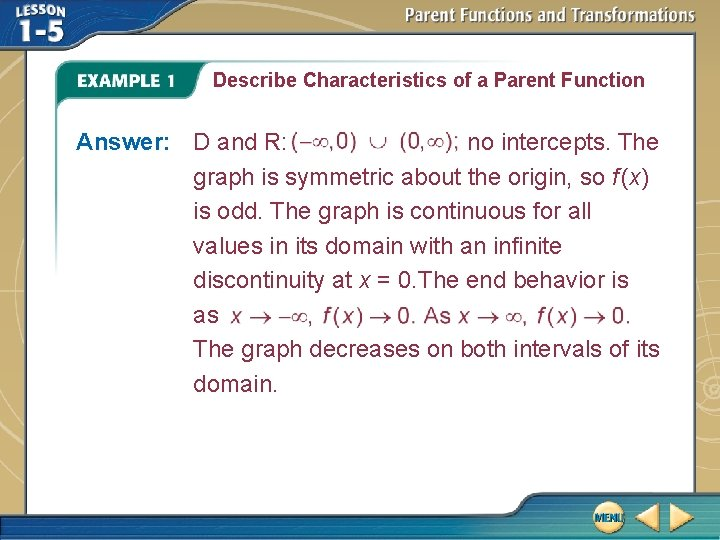Describe Characteristics of a Parent Function Answer: D and R: no intercepts. The graph is symmetric about the origin, so f (x) is odd. The graph is continuous for all values in its domain with an infinite discontinuity at x = 0. The end behavior is as The graph decreases on both intervals of its domain.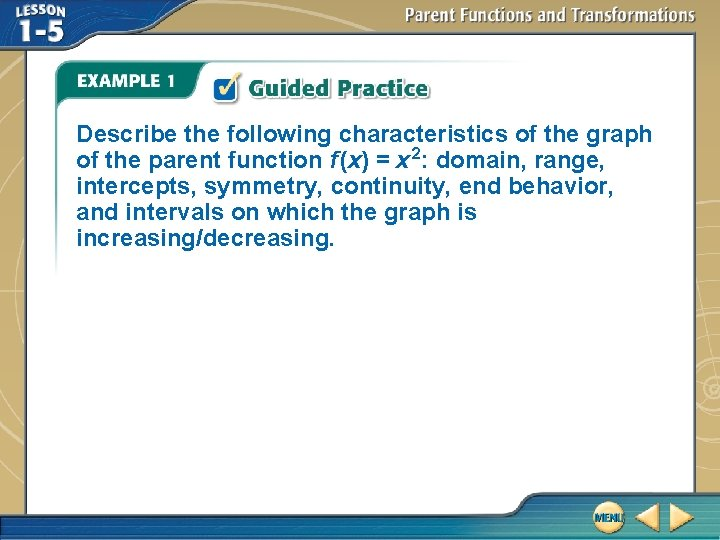Describe the following characteristics of the graph of the parent function f (x) = x 2: domain, range, intercepts, symmetry, continuity, end behavior, and intervals on which the graph is increasing/decreasing.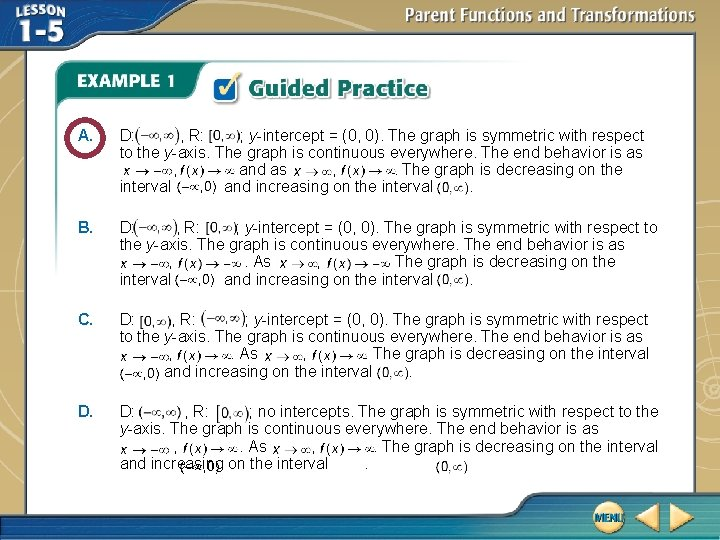A. D: , R: ; y-intercept = (0, 0). The graph is symmetric with respect to the y-axis. The graph is continuous everywhere. The end behavior is as , and as , . The graph is decreasing on the interval and increasing on the interval. B. D: , R: ; y-intercept = (0, 0). The graph is symmetric with respect to the y-axis. The graph is continuous everywhere. The end behavior is as , . As , . The graph is decreasing on the interval and increasing on the interval. C. D: , R: ; y-intercept = (0, 0). The graph is symmetric with respect to the y-axis. The graph is continuous everywhere. The end behavior is as , . As , . The graph is decreasing on the interval and increasing on the interval. D: , R: ; no intercepts. The graph is symmetric with respect to the y-axis. The graph is continuous everywhere. The end behavior is as , . As , . The graph is decreasing on the interval and increasing on the interval.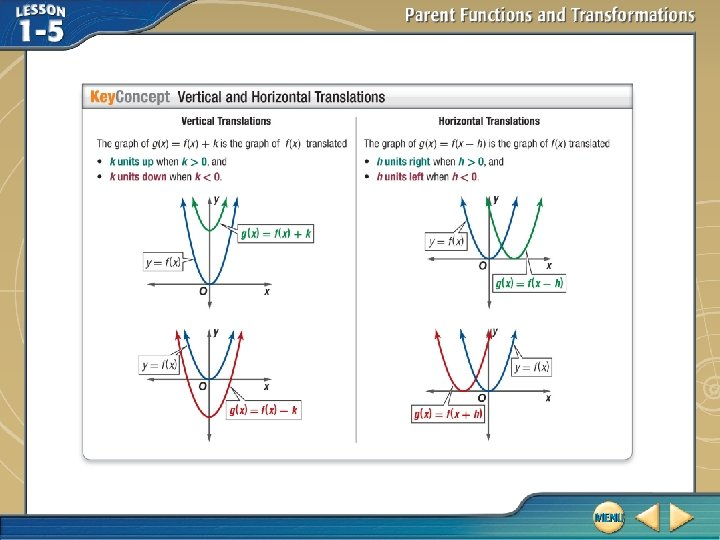Graph Translations A. Use the graph of f (x) = x 3 to graph the function g (x) = x 3 – 2. This function is of the form g (x) = f (x) – 2. So, the graph of g (x) is the graph of f (x) = x 3 translated 2 units down, as shown below. Answer: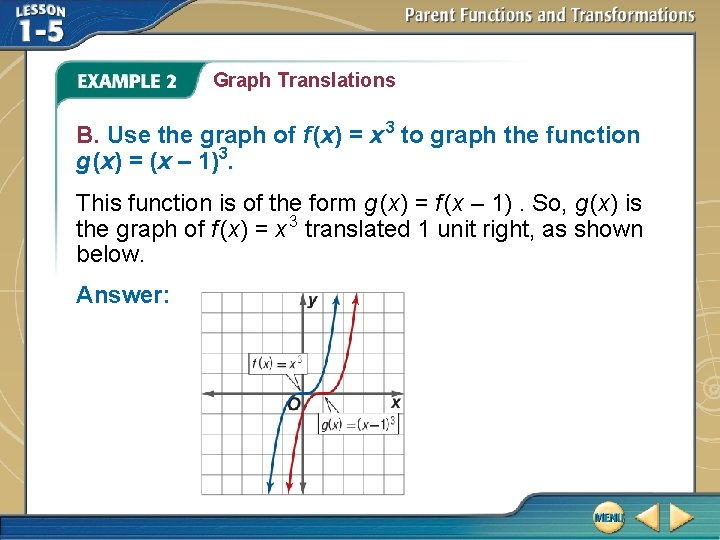Graph Translations B. Use the graph of f (x) = x 3 to graph the function g (x) = (x – 1)3. This function is of the form g (x) = f (x – 1). So, g (x) is the graph of f (x) = x 3 translated 1 unit right, as shown below. Answer:Graph Translations C. Use the graph of f (x) = x 3 to graph the function g (x) = (x – 1)3 – 2. This function is of the form g (x) = f (x – 1) – 2. So, g (x) is the graph of f (x) = x 3 translated 2 units down and 1 unit right, as shown below. Answer: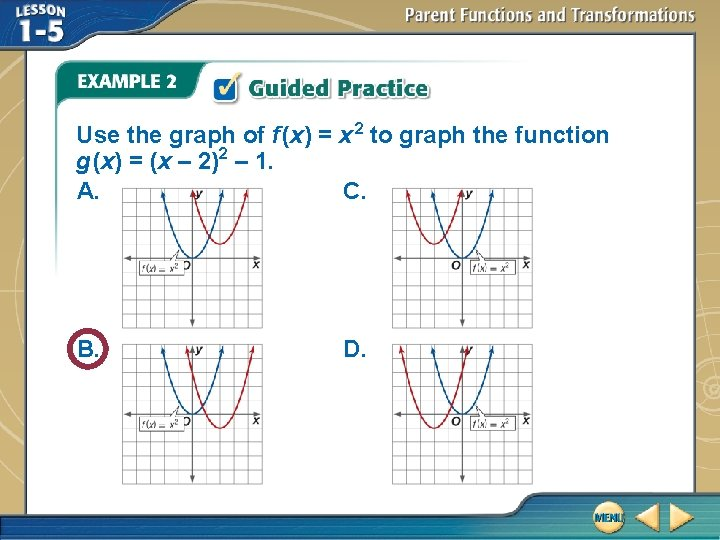Use the graph of f (x) = x 2 to graph the function g (x) = (x – 2)2 – 1. A. C. B. D.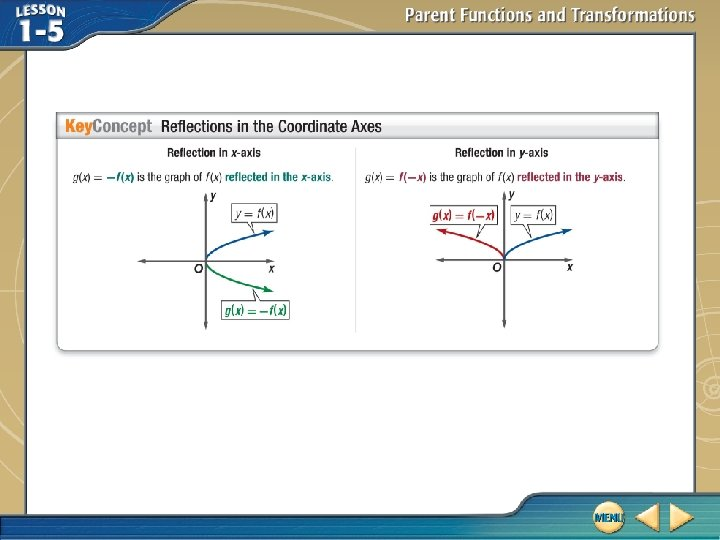Write Equations for Transformations A. Describe how the graphs of and g (x) are related. Then write an equation for g (x). The graph of g (x) is the graph of 1 unit up. So, . translated Answer: The graph is translated 1 unit up;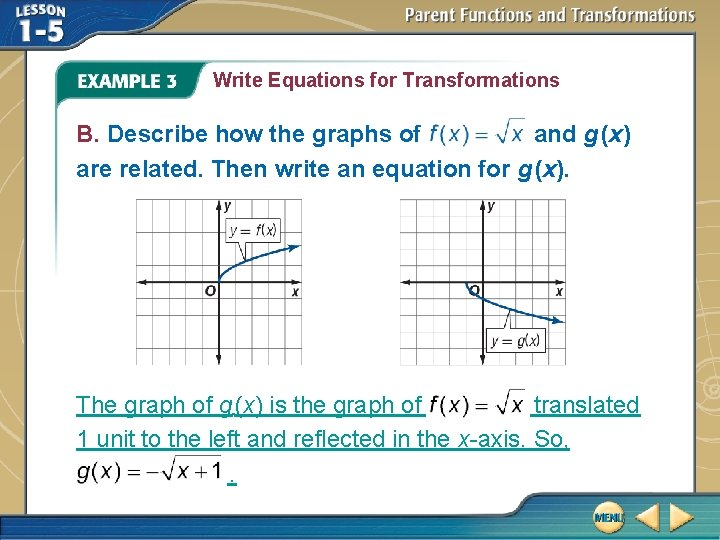Write Equations for Transformations B. Describe how the graphs of and g (x) are related. Then write an equation for g (x). The graph of g (x) is the graph of translated 1 unit to the left and reflected in the x-axis. So, .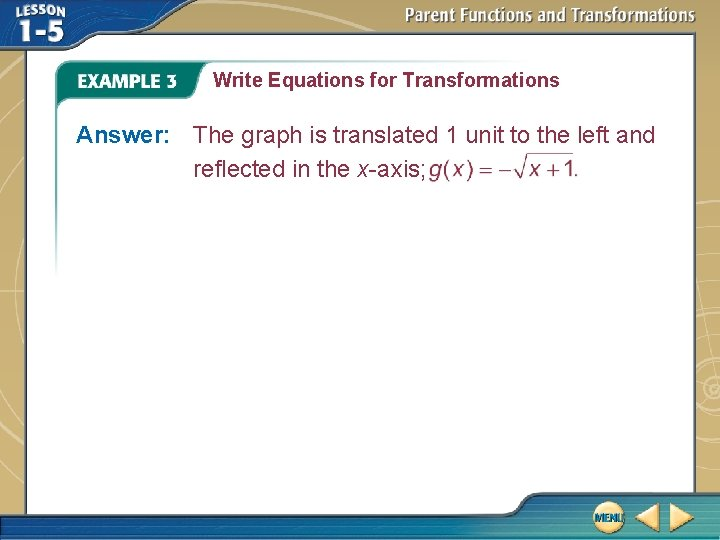Write Equations for Transformations Answer: The graph is translated 1 unit to the left and reflected in the x-axis;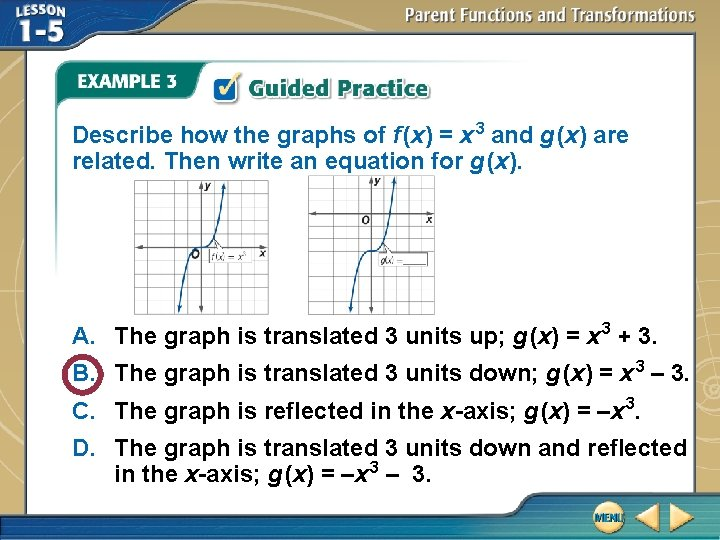Describe how the graphs of f (x) = x 3 and g (x) are related. Then write an equation for g (x). A. The graph is translated 3 units up; g (x) = x 3 + 3. B. The graph is translated 3 units down; g (x) = x 3 – 3. C. The graph is reflected in the x-axis; g (x) = –x 3. D. The graph is translated 3 units down and reflected in the x-axis; g (x) = –x 3 – 3.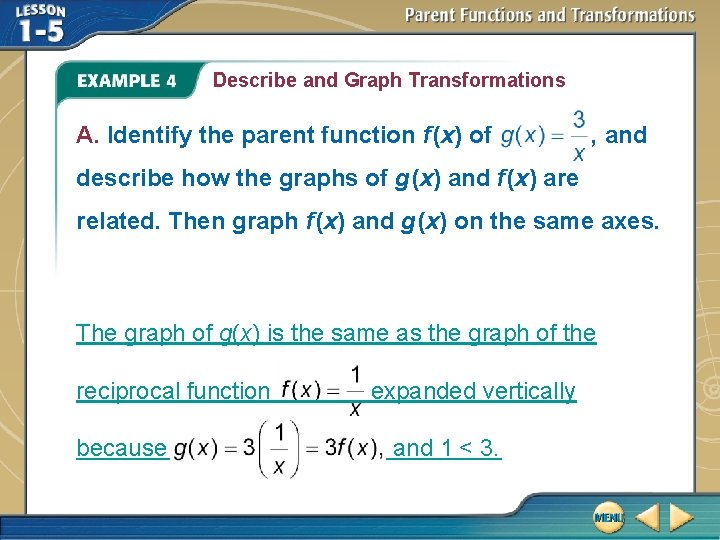Describe and Graph Transformations A. Identify the parent function f (x) of , and describe how the graphs of g (x) and f (x) are related. Then graph f (x) and g (x) on the same axes. The graph of g(x) is the same as the graph of the reciprocal function because expanded vertically and 1 < 3.Describe and Graph Transformations Answer: ; g (x) is represented by the expansion of f (x) vertically by a factor of 3.Describe and Graph Transformations B. Identify the parent function f (x) of g (x) = –|4 x|, and describe how the graphs of g (x) and f (x) are related. Then graph f (x) and g (x) on the same axes. The graph of g (x) is the same as the graph of the absolute value function f (x) = |x| compressed horizontally and then reflected in the x-axis because g (x) = – 4(|x|) = –|4 x| = –f (4 x), and 1 < 4.Describe and Graph Transformations Answer: f (x) = |x| ; g (x) is represented by the compression of f (x) horizontally by a factor of 4 and reflection in the x-axis.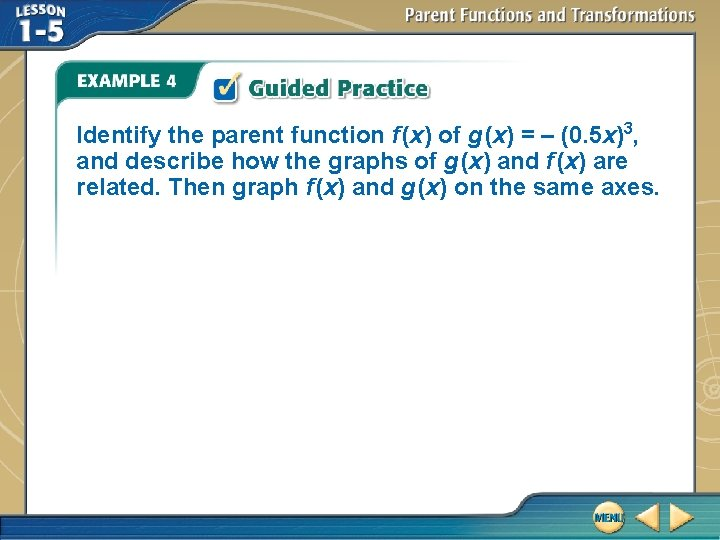Identify the parent function f (x) of g (x) = – (0. 5 x)3, and describe how the graphs of g (x) and f (x) are related. Then graph f (x) and g (x) on the same axes.A. f (x) = x 3; g(x) is C. f (x) = x 3; g(x) is represented by the expansion of the reflection of the graph of f (x) horizontally by a in the x-axis. factor of . B. f (x) = x 3; g(x) is D. f (x) = x 2; g(x) is represented by the expansion of the graph of f (x) horizontally by a factor of and reflected in the x-axis.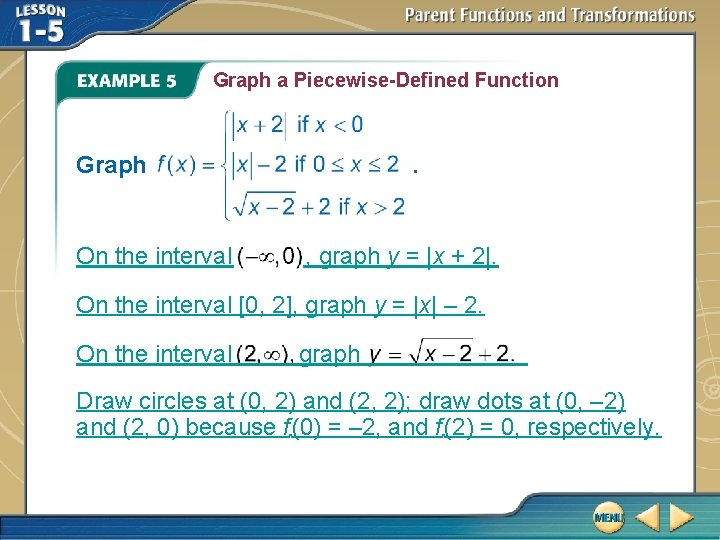Graph a Piecewise-Defined Function Graph On the interval . , graph y = |x + 2|. On the interval [0, 2], graph y = |x| – 2. On the interval graph Draw circles at (0, 2) and (2, 2); draw dots at (0, – 2) and (2, 0) because f (0) = – 2, and f (2) = 0, respectively.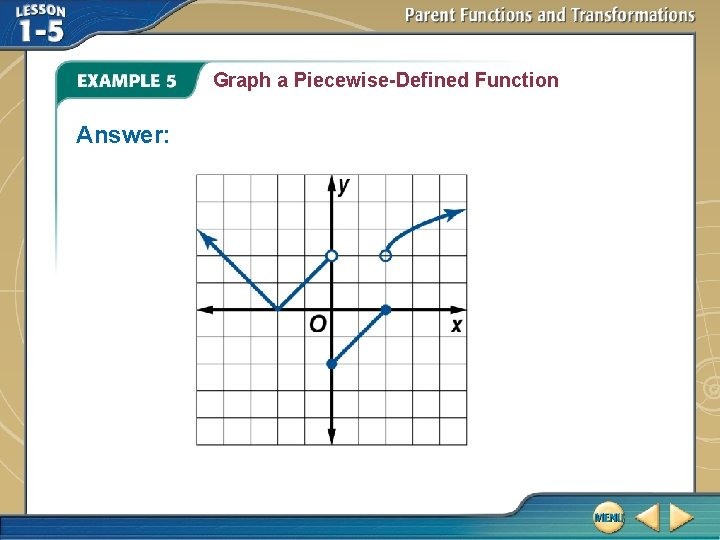Graph the function A. C. B. D. .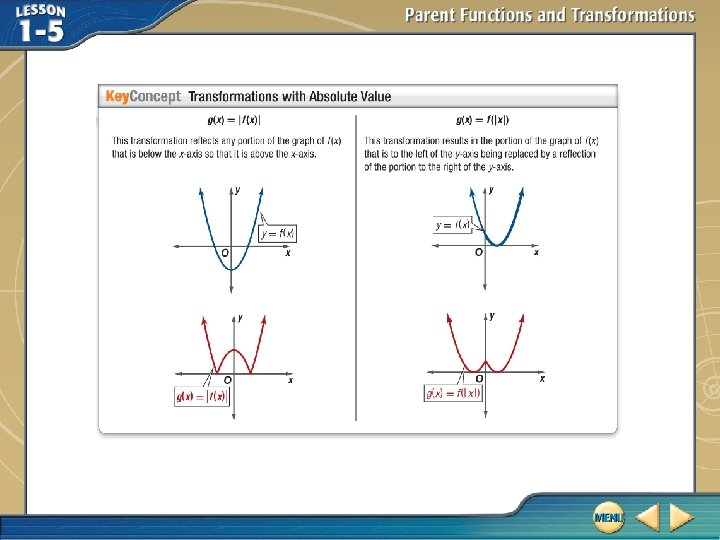Describe and Graph Transformations A. Use the graph of f (x) = x 2 – 4 x + 3 to graph the function g(x) = |f (x)|.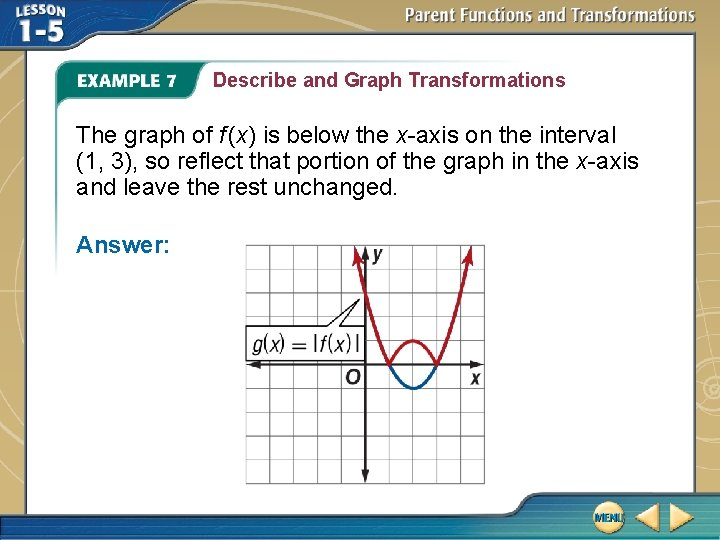Describe and Graph Transformations The graph of f (x) is below the x-axis on the interval (1, 3), so reflect that portion of the graph in the x-axis and leave the rest unchanged. Answer: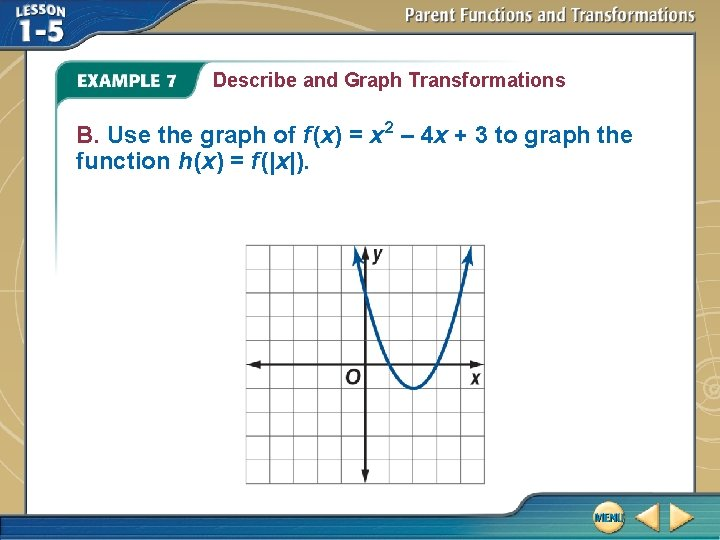Describe and Graph Transformations B. Use the graph of f (x) = x 2 – 4 x + 3 to graph the function h (x) = f (|x|).Describe and Graph Transformations Replace the graph of f (x) to the left of the y-axis with a reflection of the graph to the right of the y-axis. Answer: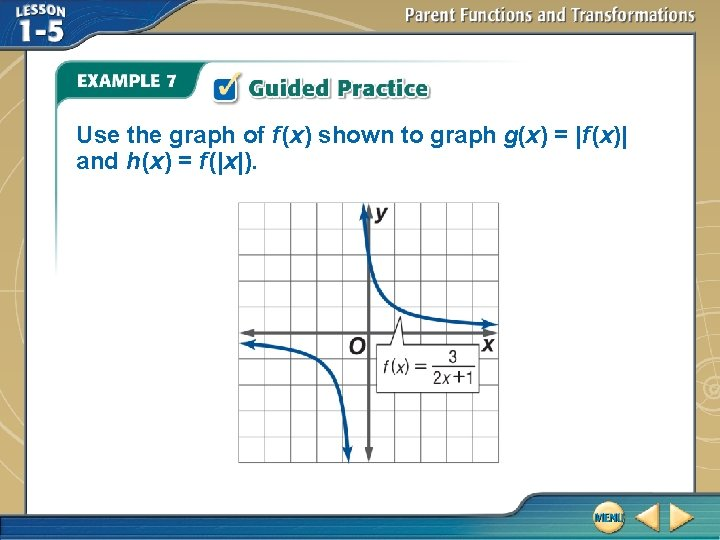Use the graph of f (x) shown to graph g(x) = |f (x)| and h (x) = f (|x|).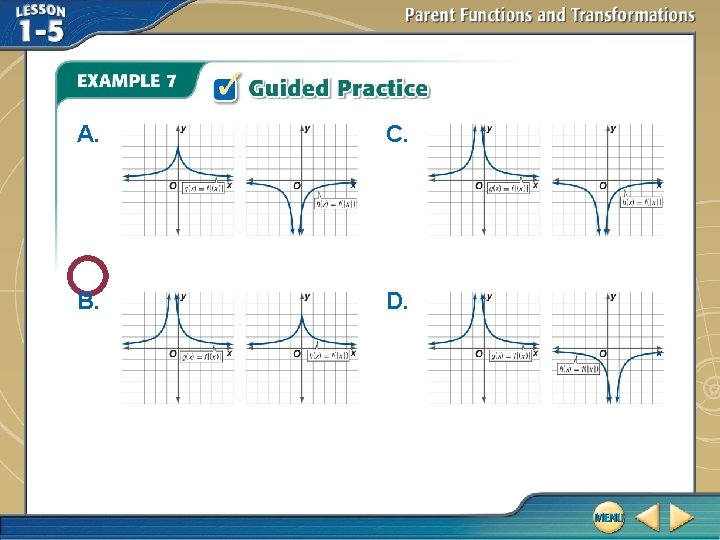A. C. B. D.